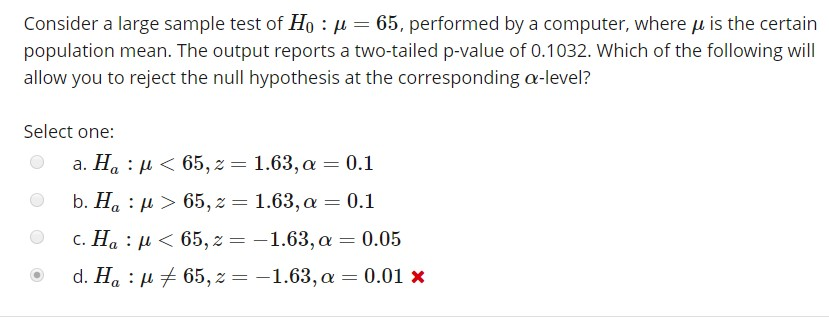# Consider a large sample test of H0 : μ-65, performed by a computer, where μ is the certainpopulation mean. The output reports a two-tailed p-value of 0.1032. Which of the following willallow you to reject the null hypothesis at the corresponding α-level?Select one:a. Ha : μ < 65,2 = 1.63, α = 0.1b. Ha : > 65,z 1.63, a 0.1c. Ha : u 65,-1.63,a-0.05

Question
1 views

Hello. I appreciate any help. Thanks.help_outlineImage TranscriptioncloseConsider a large sample test of H0 : μ-65, performed by a computer, where μ is the certain population mean. The output reports a two-tailed p-value of 0.1032. Which of the following will allow you to reject the null hypothesis at the corresponding α-level? Select one: a. Ha : μ < 65,2 = 1.63, α = 0.1 b. Ha : > 65,z 1.63, a 0.1 c. Ha : u 65,-1.63,a-0.05 fullscreen
check_circle

star
star
star
star
star
1 Rating
Step 1

We reject the Null hypothesis, when the p value of  the test statistic is less than the level of significance of the hypothesis test.

Given Null hypoth...

### Want to see the full answer?

See Solution

#### Want to see this answer and more?

Solutions are written by subject experts who are available 24/7. Questions are typically answered within 1 hour.*

See Solution
*Response times may vary by subject and question.
Tagged in

### Statistics